# How do you write text over arrows in LaTeX?

Text over arrow is an important expression, which we constantly use chemistry or in different fields. Multiple packages and commands are present to print this expression into a latex document.

\documentclass{article}
\usepackage{mathtools,halloweenmath}
\begin{document}
$\underleftarrow{abc} \; \underleftrightarrow{abcdr}\;\underrightarrow{abc}$
$\xleftarrow{chem} \; \xrightarrow{physi}$
% use of halloweenmath package
$\underscriptleftarrow{abc}\; \underscriptleftrightarrow{abc}\; \underscriptrightarrow{abc}$
\end{document}

Output :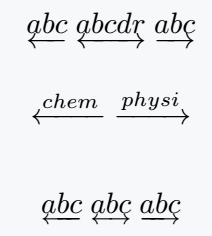amsmath package has the same command as stix package. However, in this case there are some structural changes in the shape of the arrow.

\documentclass{article}
\usepackage{stix}
\begin{document}
$\underleftarrow{pqr} \; \underleftrightarrow{pqr}\;\underrightarrow{pqr}$
$\underleftarrow{physics} \; \underleftrightarrow{math}\;\underrightarrow{chemistry}$
\end{document}

Output :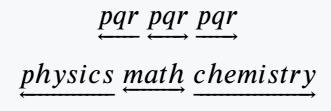You will see the use of \xrightarrow command for this expression in different latex books or online documents, which is available in the amsmath and mathtools package.

But, I think it’s not a best practices.

## Write text over double arrow in LaTeX

Notice the program below, where the \xLeftarrow and \xRightarrow commands are used for left and right double arrows.

\documentclass{article}
\usepackage{mathtools}
\begin{document}
$\xLeftarrow{use}\; \xLeftrightarrow{double} \xRightarrow{arrow}$
$\xLeftarrow{f(x)}\; \xLeftrightarrow{g(x)} \xRightarrow{f^1(x)}$
$A \xRightarrow{\Delta}\;B \xRightarrow{\Delta} C$
$\xLeftrightarrow{g^1(x)}$
\end{document}

Output :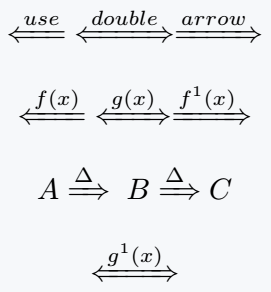However, the point is that you have used this command before, but latex is case sensitive so in both cases the spelling of the command is the same but the work will be different.

## Write text over harpoons in LaTeX

There are structural differences between harpoon and common arrow. One side of the harpoon is like an arrow, and the opposite side is like a normal line.

\documentclass{article}
\usepackage{mathtools}
\begin{document}
$\xleftharpoondown{xyz} \;\xrightharpoondown{xyz}$
$\xleftharpoonup{zyx} \;\xrightharpoonup{zyx}$
$\xleftrightharpoons{xyz}\;\xrightleftharpoons{xyz}$
\end{document}

Output :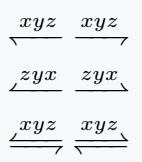## Write text above and below arrow in LaTeX

If you want to use text at the above and below of an arrow at the same time, the chemarrow package is best practice. For example

\documentclass{article}
\usepackage{chemarrow}
\begin{document}
$A + B \; \autorightarrow{m}{h}\;C$
$D \; \autoleftarrow{a}{h}\;M + N$
$A + B \;\autorightleftharpoons{m}{h}\; C + D$
\end{document}

Output :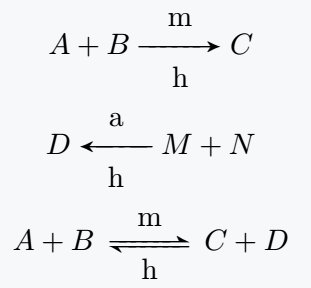You can add text under each arrow bypassing the text as an optional argument with each text over arrow command in the mathtools package.

\documentclass{article}
\usepackage{mathtools}
\begin{document}
$\xleftarrow[qrs]{pqr} \; \xrightarrow[qrs]{pqr}$
$\xhookleftarrow[pqr]{qrs} \; \xhookrightarrow[pqr]{qrs}$
$\xLeftarrow[pqr]{abc} \; \xRightarrow[pqr]{abc}$
$B + HNO_3\;\xrightarrow[\Delta]{H_2SO_4}\; H_3BO_3 + NO_2$
\end{document}

Output :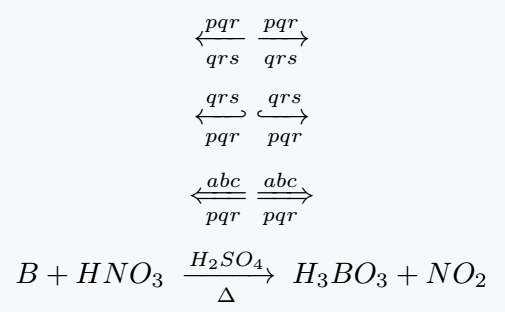#### Md Jidan Mondal

LaTeX expert with over 10 years of experience in document preparation and typesetting. Specializes in creating professional documents, reports, and presentations using LaTeX.